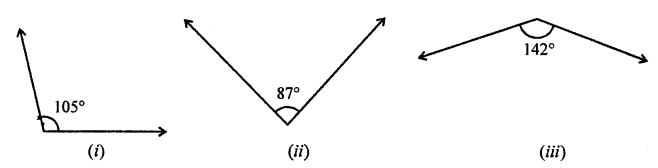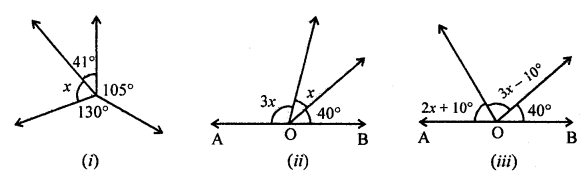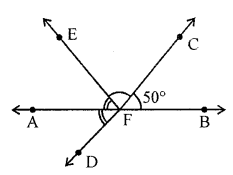## ML Aggarwal Class 7 Solutions for ICSE Maths Chapter 10 Lines and Angles Ex 10.1

Question 1.
(i) Can two right angles be complementary?
(ii) Can two right angles be supplementary?
(iii) Can two adjacent angles be complementary?
(iv) Can two adjacent angles be supplementary?
(v) Can two obtuse angles be adjacent?
(vi) Can an acute angle be adjacent to an obtuse angle?
(vii) Can two right angles form a linear pair?
Solution:
(i) No, as the sum of two complementary angles is 90°.
(ii) Yes.
(iii) Yes.
(iv) Yes.
(v) Yes.
(vi) Yes.
(vii) Yes.

Question 2.
Find the complement of each of the following angles:Solution:
(i) Complement of 25° = 90° – 25° = 65°
(ii) Complement of 63° = 90° – 63° = 27°
(iii) Complement of 57° = 90° – 57° = 33°

Question 3.
Find the supplement of each of the following angles:Solution:
(i) Supplement of 105° is 180° – 105° = 75°
(ii) Supplement of 875° is 180° – 87° = 93°
(iii) Supplement of 142° is 180° – 142° = 38°

Question 4.
Identify which of the following pairs of angles are complementary and which are supplementary:
(i) 55°, 125°
(ii) 34°, 56°
(iii) 137°, 43°
(iv) 112°, 68°
(v) 45°, 45°
(vi) 72°, 18°
Solution:
(i) 55°, 125° are supplementary.
(ii) 34°, 56° are complementary.
(iii) 137°, 43° are supplementary.
(iv) 112°, 68° are supplementary.
(v) 45°, 45° are complementary.
(vi) 12°, 18° are complementary.

Question 5.
(i) Find the angle which is equal to its complement.
(ii) Find the angle which is equal to its supplement.
Solution:
(i) $$\frac { 90 }{ 2 }$$ = 45°
45° is complement to 45°
(ii) $$\frac { 180 }{ 2 }$$ = 90°
90° is supplement to 90°

Question 6.
Two complementary angles are (x + 4)° and (2x – 7)°, find the value of x.
Solution:
(x + 4)° and (2x – 7)° are complementary angles
x + 4 + 2x – 7 = 90°
⇒ 3x – 3 = 90°
⇒ 3x = 90° + 3 = 93°
x = 31°

Question 7.
Two supplementary angles are in the ratio of 2 : 7, find the angles.
Solution:
Two supplementary angles in the ratio 2 : 7 and sum is 180°Question 8.
Among two supplementary angles, the measure of the longer angle is 44° more than the measure of the smaller angle. Find their measures.
Solution:
Sura of two supplementary angles = 180°
Let smaller angle = x Then second angle = x + 44°
x + x + 44° = 18°
⇒ 2x = 180° – 44° = 136°
⇒ 2x = 136°
⇒ x = 68°
One angle = 68°
and second angle = 68° + 44° = 112°

Question 9.
If an angle is half of its complement, find the measure of angles.
Solution:
Sum of complementary angles = 90°
Let on angle = x°
Then second angle = $$\frac { x }{ 2 }$$
x + $$\frac { x }{ 2 }$$ = 90°
⇒ $$\frac { 3x }{ 2 }$$ = 90°
⇒ 3x = 90° × 2 = 180°
⇒ x = 60°
One angle = 60°
and the other angle = $$\frac { 60 }{ 2 }$$ = 30°
Angles are 30°, 60°

Question 10.
Two adjacent angles are in the ratio 5 : 3 and they together form an angle of 128°, find these angles.
Solution:
Sum of two adjacent angles = 128°
and ratio between them = 5 : 3
Let first angle = 5x
Then second angle = 3x
5x + 3x = 128°
⇒ 8x = 128°
⇒ x = 16°
First angle = 5x = 5 × 16° = 80°
and second angle = 3x = 3 × 16 = 48°

Question 11.
Find the value of x in each of the following diagrams:Solution:
In the given figure,
(i) 105° + 41° + x° + 130° = 360°
(Angles at a point)
⇒ 276° + x = 360°
⇒ x = 360° – 276° = 84°
⇒ x = 84°
(ii) In the given figure,
3x + x + 40° = 180°
(Angles on one side of a straight line)
⇒ 4x = 180° – 40° = 140°
⇒ x = 35°
(iii) In the given figure,
2x+ 10 + 3x – 10 + 40 = 180°
(Angles on one side of a straight line)
⇒ 5x + 40° = 180°
⇒ 5x = 180° – 40° = 140°
⇒ x = 28°

Question 12.
Find the values of x, y and z in each of the following diagrams:Solution:
(i) In the given figure,
Given ∠ = 135°
y = 135° (Vertically opposite angles)
But x + y = 180° (Linear pair)
⇒ x + 135° = 180°
⇒ x = 180° – 135° = 45°
But z = x (Vertically opposite angles)
z = 45°
Hence, x = 45°, y = 135°, z = 45°
(ii) In the given figure,
Given ∠ = 31°
x = 31° (Vertically opposite angles)
But 31° + y + 90° = 180°
(Angles on one side of a straight line)
⇒ y + 121° = 180°
⇒ y = 180° – 121° = 59°
But z = y (Vertically opposite angles)
z = 59°
Hence, x = 31°, y = 59°, z = 59°
(iii) In the given figure,
Given ∠s are 51° and 44°
Let ∠1 = 44° and ∠2 = 51°
x = 44° (Vertically opposite angles)
and ∠2 = ∠z, z = 51° (Vertically opposite angles)
∠1 = ∠x
But x + y + z = 180° (Angles on one side of a line)
⇒ 44° + 51° + y= 180°
⇒ 95° + y = 180°
⇒ y = 180° – 95° = 85°
y = 85°, z = 51°
Hence, x = 44°, y = 85°, z = 51°

Question 13.
In the given figure, lines AB and CD intersect at F. If ∠EFA = ∠AFD and ∠CFB = 50°, find ∠EFC.Solution:
In the given figure,AB and CD intersect each other at F.
∠EFA = ∠AFD and ∠CFB = 50°
To find : ∠EFC
∠AFD = ∠BFC  = 50° (Vertically opposite angles)
But ∠EFA = ∠AFD (given) = 50°
Now, ∠EFA + ∠EFC + ∠CFB = 180°
(Angles on one side of straight line)
⇒ 50° + ∠EFC + 50° = 180°
⇒ ∠EFC + 100° = 180°
⇒ ∠EFC =180° – 100° = 80°
⇒ ∠EFC = 80°